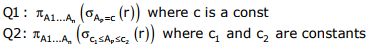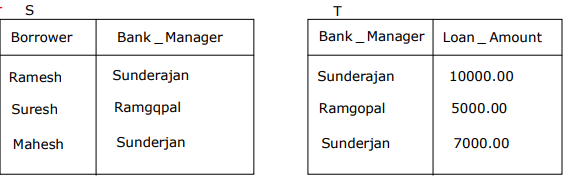# Databases - Online Test

Q1. What is the chromatic number of an n-vertex simple connected graph which does not contain any odd length cycle? Assume n ≥ 2
Explaination / Solution:
No Explaination.

Q2. Which of the following statement (s) is/are correct regarding Bellman-Ford shortest path algorithm? P. Always find a negative weighted cycle, if one exists. Q. Finds whether any negative weighted cycle is reachable from the source.
Explaination / Solution:
No Explaination.

Q3. Let R and S be relational schemes such that R={a, b, c} and S={c}. Now consider the following queries on the database:Where R.c = S.c
Which of the above queries are equivalent?
Explaination / Solution:
No Explaination.

Q4. Consider the following relational schema: Suppliers (sid:integer, sname: string, city:string, street: string) Parts (pid:integer, pname: string, color:string) Catalog (sid:integer, pid:integer, cost: real)
Consider the following relational query on the above database:
SELECT S.sname
FROM Suppliers S
WHERE S.sid NOT IN (SELECT C. sid
FROM Catalog C
WHERE C.pid NOT IN (SELECT P.pid
FROM Parts P
WHERE P.color<> ‘blue’))
Assume that relations corresponding to the above schema are not empty. Which one of the following is the correct interpretation of the above query?
Explaination / Solution:
No Explaination.

Q5. Consider the following relational schema: Suppliers (sid:integer, sname: string, city:string, street: string) Parts (pid:integer, pname: string, color:string) Catalog (sid:integer, pid:integer, cost: real) Assume that, in the suppliers relation above, each supplier and each street within a city has a unique name, and (sname, city) forms a candidate key. No other functional dependencies are implied other than those implied by primary and candidate keys. Which one of the following is TRUE about the above schema?
Explaination / Solution:
No Explaination.

Q6.
Two alternative packages A and B are available for processing a database having 10k records. Package A requires 0.0001ntime units and package B requires 10nlog10n time units to process n records. What is the smallest value of k for which package B will be preferred over A?
Explaination / Solution:
No Explaination.

Q7. Consider a relational table with a single record for each registered student with the following attributes. 1. Registration_Number: Unique registration number for each registered student 2. UID: Unique Identity number, unique at the national level for each citizen 3. BankAccount_Number: Unique account number at the bank. A student can have multiple accounts or joint accounts. This attributes stores the primary account number 4. Name: Name of the Student 5. Hostel_Room: Room number of the hostel Which of the following options is INCORRECT?
Explaination / Solution:

In case two students hold joint account then BankAccount_Num will not uniquely determine other attributes.

Q8. Consider a relational table r with sufficient number of records, having attributes A1, A2,…, An and let 1 ≤ p ≤ n. Two queries Q1 and Q2 are given below.The database can be configured to do ordered indexing on Ap or hashing on Ap
Which of the following statements is TRUE?
Explaination / Solution:
No Explaination.

Q9. Database table by name Loan_Records is given below.
Borrower          Bank_Manager                    Loan_ Amount
Ramesh              Sunderajan                           10000.00
Suresh                Ramgopal                             5000.00
Mahesh              Sunderajan                            7000.00
What is the output of the following SQL query?
SELECT count(*)
FROM(
(SELECT Borrower. Bank_Manager FROM Loan_Records) AS S
NATURAL JOIN
(SELECT Bank_Manager, Loan_Amount FROM Loan_Records) AS T
);
Explaination / Solution:After executing the given query, the output would beQ10. Consider a database table T containing two columns X and Y each of type integer. After the creation of the table, one record (X= 1, Y=l) is inserted in the table. Let MX and MY denote the respective maximum values of X and Y among all records in the table at any point in time. Using MX and MY, new records are inserted in the table 128 times with X and Y values being MX+1, 2*MY+1 respectively. It may be noted that each time after the insertion, values of MX and MY change. What will be the output of the following SQL query after the steps mentioned above are carried out? SELECT Y FROM T WHERE X=7;
Explaination / Solution: